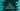# C# program to check if a number is Strong number or not## C# program to check if a number is Strong number or not:

A number is called a Strong number if the sum of all factorials of the digits in the number is equal to the number. For example, 145 is a strong number because 1! + 4! + 5! = 145. In this post, we will learn how to check if a user given number is strong or not in C#.

We will write one C# program that will take one number as input from the user and it will print one message that the number is strong number or not.

### C# program:

Below is the complete C# program:

``````using System;

public class Program
{
static int getFactorial(int number){
int factorial = 1;
for(int i = number; i > 1; i--){
factorial = factorial * i;
}
return factorial;
}

public static void Main()
{
int givenNumber;

Console.WriteLine("Enter a number to check");

int sum = 0;
int copyNumber = givenNumber;

while(copyNumber != 0){
int lastDigit = copyNumber%10;
sum = sum + getFactorial(lastDigit);
copyNumber = copyNumber / 10;
}

if(sum == givenNumber){
Console.WriteLine("Given number is a strong number");
}else{
Console.WriteLine("Given number is not a strong number");
}

}
}``````

### Explanation:

Here,

1. givenNumber is an integer variable to hold the user input number. We are reading one number that user entered on console and storing it in givenNumber.
2. sum variable is used to hold the sum of all factorials of the digits of a number.
3. We are copying the value of user entered number to copyNumber so that we don’t have to modify the original variable.
4. Using a while loop, we are getting the factorial of each digit and adding that value to sum. lastDigit is the last digit and we are finding it using number % 10. getFactorial method returns the factorial of a number that we are passing. At the end of the while loop, we are changing the number to number / 10, i.e. we are removing the last digit from that number.
5. The while loop runs until the value of copyNumber become 0.
6. After the while loop ends, we are checking if sum is equal to givenNumber or not. If equal, we are printing that the given number is strong, else not.

### Sample Output:

If you run this program, it will print outputs as like below :

``````Enter a number to check
145
Given number is a strong number

Enter a number to check
10
Given number is not a strong number``````